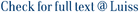We study various partially ordered spaces of probability measures and we determine which of them are lattices. This has important consequences for optimization problems with stochastic dominance constraints. In particular we show that the space of probability measures on \$\mathbb{R}\$ is a lattice under most of the known partial orders, whereas the space of probability measures on \$\mathbb{R}^d\$ typically is not. Nevertheless, some subsets of this space, defined by imposing strong conditions on the dependence structure of the measures, are lattices.

Stochastic order relations and lattices of probability measures / Mueller, A.; Scarsini, Marco. - In: SIAM JOURNAL ON OPTIMIZATION. - ISSN 1052-6234. - 16:(2006), pp. 1024-1043. [10.1137/040611021]

### Stochastic order relations and lattices of probability measures

#### Abstract

We study various partially ordered spaces of probability measures and we determine which of them are lattices. This has important consequences for optimization problems with stochastic dominance constraints. In particular we show that the space of probability measures on \$\mathbb{R}\$ is a lattice under most of the known partial orders, whereas the space of probability measures on \$\mathbb{R}^d\$ typically is not. Nevertheless, some subsets of this space, defined by imposing strong conditions on the dependence structure of the measures, are lattices.
##### Scheda breve Scheda completa Scheda completa (DC)2006
Stochastic order relations and lattices of probability measures / Mueller, A.; Scarsini, Marco. - In: SIAM JOURNAL ON OPTIMIZATION. - ISSN 1052-6234. - 16:(2006), pp. 1024-1043. [10.1137/040611021]
File in questo prodotto:
File
3 - sjo2006.pdf

Solo gestori archivio

Tipologia: Documento in Post-print
Licenza: DRM (Digital rights management) non definiti
Dimensione 307.39 kB
Utilizza questo identificativo per citare o creare un link a questo documento: `https://hdl.handle.net/11385/3152`
•21
•22